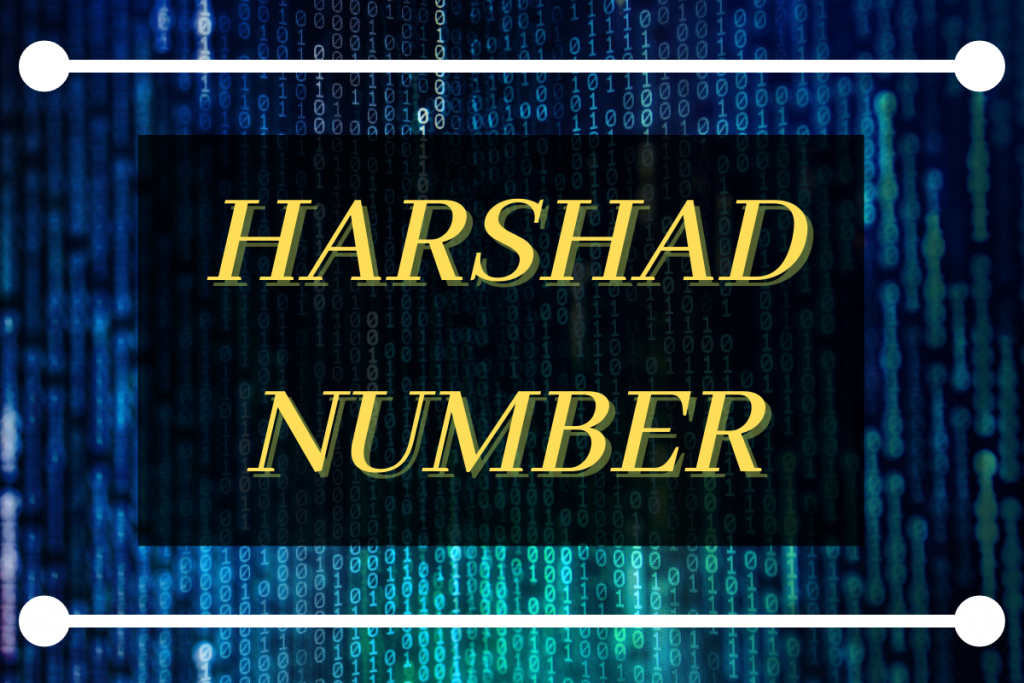# Harshad Number in Python – Easy ImplementationHello there! Today let us learn about Harshad Number. We would be understanding what the number is and then implement a program to check if a number is a Harshad Number or not.

## What is a Harshad Number?

A number is a Harshad number if the original number is divisible by the sum of its digits.

Harshad Number definition is : abcd is divisible by (a+b+c+d).

Recommended read: How to check for Armstrong number in Python?

### Example 1: 155

Sum of the digits = 1 + 5 + 5 = 11

But 155 is not divisible by 11. Hence the number is not a Harshad number.

### Example 2: 156

Sum of the digits = 1 + 5 + 6 = 12

But 156 is divisible by 12. Hence the number is a Harshad number.

## Algorithm to check Harshad Number

All the steps involved in checking if a number is a Harshad number or not are:

2. Make a copy of the number to check the result later on.
3. Create a result variable ( set to 0 )
4. Create a while loop to traverse through the number digit by digit.
5. On every iteration increment result by digit
6. Divide the copy of the number by the result obtained.
7. If it divides perfectly then a number is a Harshad Number otherwise it isn’t.

The code below shows the pseudo code to check if a number is a Harshad Number:

```READ n
MAKE A COPY OF n
result=0
CHECK DIGIT BY DIGIT:
WHILE n!=0
GET CURRENT DIGIT : digit = n % 10
UPDATE RESULT : result = result + digit
TRIM THE LAST DIGIT : n = n / 10
ENDWHILE

IF COPY OF n % result == 0
ELSE
```

## Code to Check Harshad Number in Python

Now that we know about what the Harshad Number is and the steps to implement that, let’s implement the Harshad checking line by line.

### Create the intital variables

We first take an input `n` and store a copy of the input so that no matter how much we change the original number We also initialized the result as 0.

The code for the same is shown below:

```n = input()
n=int(n)
copy_n=n
result = 0
```

### Traversing through the number and Updating Result

To access each digit we take the `modulus` of the number ( mod 10 ) to extract the last digit of the number. The next step involves updating the result as the sum of the previous result and the current digit.

The last and final step we take is to divide the number by 10 to drop the last digit from the number. The same process is repeated until there are no more digits are left in the number.

The code for the same is shown below:

```while(n!=0):
digit = n%10
result=result + digit
n=int(n/10)
```

### Checking if the number is a Harshad Number or not

The final step is to check if the copy of the number we created earlier is divisible by the result calculated or not. The code for the same is shown below:

```if(copy_n % result == 0):
else:
```

## Output Samples for the code

For now I tested the program for two inputs. Both outputs are shown below:

```156
```121